# Reason - 8th grade (13y) - math problems

#### Number of problems found: 473

• Seven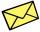Seven friends agree to send everyone a holiday card. How many postcards were sent?
• Coins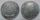On coins found was stated that from the year 87 BC. It is right?
• One threeWe throw two dice. What is the probability that max one three falls?
• Pair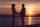They have together 39 years - he has 2 times as she was when he was equal as she has today ....
• Two integers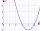Two integers, a and b, have a product of 36. What is the least possible sum of a and b?
• ProbabilityHow probable is a randomly selected three-digit number divisible by five or seven?
• DivisibilityWrite all the integers x divisible by seven and eight at the same time for which the following applies: 100
• BracketsPlace one pair of brackets in expression 20-16 + 10 - 4 - 2 so that the result will be 0.
• Chess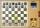How many different ways can initiate a game of chess (first pass)?
• The diceWhat is the probability of events that if we throw a dice is rolled less than 6?
• Two-element combinations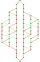Write all two-element combinations from elements a, b, c, d.
• Tricolors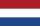From the colors - red, blue, green, black and white, create all possible tricolors.
• Probably memberLook at the series 2,6,25,96,285, ? What number should come next?
• YearsSusan is 3 years and as she will be old as Teodor now him will be 11. How old is Teodor today?
• Marriage sttusIn our city, there are 3/5 of the women married to 2/3 of the men. Find what part of the population is free.
• Combinations of sweatersI have 4 sweaters two are white, 1 red and 1 green. How many ways can this done?
• Three ints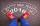The sum of three consecutive integers is 2016. What numbers are they?
• Two ages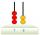Gabrielle is 9 years younger than Mikhail. The sum of their ages is 87. What is Mikhail's age?
• FamilyMom is 42 years old and her daughters 13 and 19. After how many years will mother as old as her daughter together?
• Series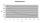Andrew wrote this series of numbers: 1,3,7,15, .... n. Which number in the series does not belong? ?

Do you have an interesting mathematical word problem that you can't solve it? Submit a math problem, and we can try to solve it.

We will send a solution to your e-mail address. Solved examples are also published here. Please enter the e-mail correctly and check whether you don't have a full mailbox.

Please do not submit problems from current active competitions such as Mathematical Olympiad, correspondence seminars etc...